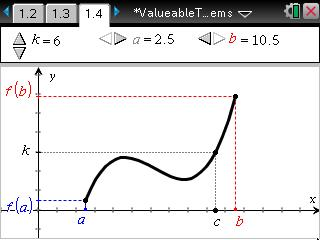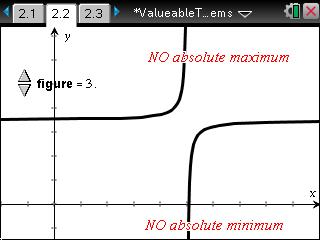# Activities

•##### Download

•• ##### Subject Area

• Math: Calculus: Derivatives
• Math: Calculus: Other Functions

• ##### Author9-12

15 Minutes

• ##### Device
•TI-Nspire™ CX/CX II
•TI-Nspire™ CX CAS/CX II CAS
• TI-Nspire™ CAS
• ##### Software

TI-Nspire™ CAS

3.0

• ##### Report an Issue

"Value"able Theorems

#### Activity Overview

This activity is a demonstration of the Intermediate Value Theorem and the Extreme Value Theorem from a graphical perspective.

#### Key Steps

•It is stated that f(x) is a continuous function on a finite closed interval [a, b]. The teacher can use a slider to change the value of k and graphically demonstrate that there is a value c on the interval [a, b] such that f(c) = k.

•For the Extreme Value Theorem, three figures are given showing an example and two non-examples of the theorem.